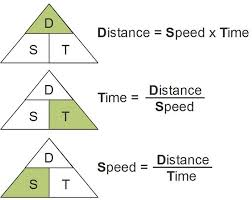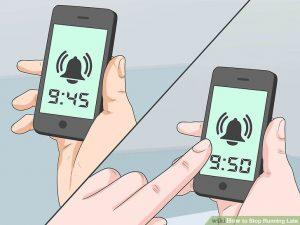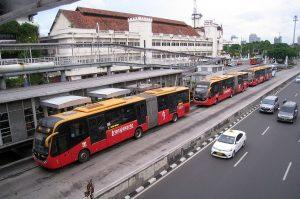# How to solve Time and Distance Questions?

Hi Bankersdaily Aspirant,

Here we are launching a New Series called  “Learn Series” which is helpful for the beginners who are preparing for Competitive Examinations like IBPS PO, SBI PO and other placement programs. We will Publish a new topic on Everyday. We explain Each and Every concept related to the topic. Read it and if you have any further queries, comment below in the comment box.

### An important key to self-confidence is preparation.”

Dear Aspirant it is right time to start preparation for the IBPS Exam and Solving Aptitude is not that much tough as we consider So,today let us see what are all the possible questions asked in  Time and Distance and shortcuts to solve the question

### INTRODUCTION:

Time is referred as time taken to complete a certain distance and its unit is hr/min/sec, Speed is referred as the rate at which a certain distance is covered and its unit is km/hr,m/sec , Distance is referred as the total distance covered its unit is km/m.

Time and Distance Formulas

Distance=Speed*Time(km/m)

Speed=Distance/Time(km/hr (or) m/sec)

Time=Distance/Speed(hr/sec)

 SPEED TIME DISTANCE Km/hr Hr km m/sec Sec m

### AVERAGE SPEED WHEN DISTANCE ARE SAME :

Average Speed = Total Distance/Total Time

Average Speed (when distance is same and there is 2 speed) =2xy/(x+y)

Average Speed (when distance is same and there is 3 speed) =3xyz/(xy+yz+zx)

### TYPE CONVERSIONS:

If the unit is in km/hr and it is converted into m/sec by multiplying it with 5/18,

If the unit is in m/sec and it is converted into km/hr by multiplying it with 18/5,

1 km/hr  is converted into 1m/sec by *(5/18)

1 m/sec is converted into 1 Km/hr by *(18/5)

### PROPORTIONAL:

If Distance is Constant then, Time(T1 &T2) and Speed(S1 and S2) is inversely proportional to each other S1/S2=T2/T1

If Time is Constant then, the ratio between Distance and Speed is directly proportional to each other.

(i.e. If Speed increases then Distance is also increases,If Speed decreases then Distance also decreases)

## #1 TYPE 1: BASED ON TIME ,DISTANCE AND SPEED:If a man rides a distance of 540km at the rate of 60Km/hr .Then at what speed he should ride in order to reach the destination less than 3 hrs than his usual speed express in m/sec?

Time=Distance/Speed

=540/60

=9hr.

Speed=Distance/Time(time =(9-3hr)=6hr)

=540/6

=90km/hr.

Type Conversion:

1 Km/hr ⇒ 1m/sec *(5/18)

90km/hr=90*(5/18)=25m/sec

### Try & Practice Questions:

1. A increases his speed by 5km/hr then time taken reduced by 20%.What is the initial speed of journey?

a) 10km/hr b) 15km/hr c) 20km/hr d) 25km/hr

2.A Car travelling with 5/7 of the actual speed covers 42km in 1hr 40min 48sec.Find the actual speed of the car?

a) 17km/hr b) 32km/hr c) 31km/hr d) 35km/hr

3. A man travels on a Scooter from A to B at a speed of 30km/hr and returns back from B to A at 20km/hr. The total journey was performed by him in 10hr.Find the distance from A to B?

a) 100km b) 110km c) 120km d) 125km

## #2 TYPE 2 : BASED ON REACHING THE DESTINATION EARLY AND LATE:If a man walks from home to his office at the rate of 40km/hr and he is late by 20 minutes and if man goes to his office from home by riding a bike at the rate of 60km/hr then he reaches the office 10 minutes early. Then what is the distance between his home and his office?

Solution:

Let us consider a man starts from his home by 9.00A.M.

By walking he would reach at 9.20 A.M.

By Riding he would reach at 8.50 A.M.

Here we don’t know the distance between them so lets take L.C.M of speed and consider it as the total distance

L.C.M. of 40,60=60km

By walking a man takes 60/40=1hr 30 mins

By Riding a man takes 60/60=1hr.

The difference between the time taken here is 30 mins(1hr 30 mins and 1hr is 30 mins)

The difference between 8.50 AM -9.20 AM=30 minutes.

Here the difference between the time taken here is 30 mins(1hr 30 mins and 1hr is 30 mins) and The difference between 8.50 AM – 9.20 AM=30 minutes is same.

Therefore the distance between his home and office is 60Km.

Shortcut Method:

LCM of 40,60=60 KM

By Walking he takes =60/40=1hr 30 min

By Riding he takes=60/60=1 hr

1hr30Min-1hr=30 Min

8.50 A.M. – 9.20 A.M.=30 Mins

Here they are same (30 Mins=30 Mins) So the distance is 60 Km

TRY & Practice Questions:

1)Starting from his house one day ,a student walks at a speed of 2(1/2)kmph and reaches his school 6minutes late .Next day increases his speed by 1kmph and reaches the school 6 minutes early. How far the is the school from his house?

a)1km b)15km c)14km d)1(3/4)km

2) If I walk at 4km/hr, I miss the bus by 10 min. If I walk at 5km/hr, I reach 5 min before the arrival of the bus. How far I walk to reach the bus stand?

a)5km b)5.5km c)6km d)7.5km

3.If a man goes to his office by bicycle from home at the rate of 60km/hr he reaches 10 minutes early and If a man goes to his office by bike from home at the rate of 80km/hr then he reaches the office 25 minutes early so what is the distance between the his home and his office?

A) 60km B) 200km C) 300km D) 400km

## #3 TYPE 3: BASED ON AVERAGES:

### For Different Distance:

Average Speed=(Total distance/Total Time Taken)

For Same Distance :

 Number Of Speed given Average Speed 2 Speed namely x and y 2xy/(x+y) 3 Speed namely x,y and z 3xyz/(xy+yz+zx)

1.A bus moves along the road which is in the shape of square.It’s speed along each side of the square are recorded as 50km/hr,25km/hr,60km/hr and 90km/hr .Find the approximate average speed of the bus in km/hr)?

Solution:

Here we don’t know the distance  bus moves so, take

LCM(50,25,60,90=900km)

But here they say the Bus moves in a square path so the distance covered by the bus=900*4=3600.

Average Speed=Total Distance /Total Time

Time taken in 1st path=900/50=18hr

Time taken in 2nd path=900/25=36hr

Time taken in 3rd path=900/60=15hr

Time taken in 4th path=900/90=10hr

Average Speed=3600/(18+36+15+10)=3600/79=45km/hr

2 .A man walks 7.5 km at a speed of 3km/hr. At what speed would the man need walk during the next 2hr to have an average of 4km/hr for the entire session?

Solution

Time=Distance/Speed

=7.5/3  ⇒ 2hr30min.

 Speed Time Distance 3 km/hr 2hr 30min 7.5km X km/hr 2hr 2X Average Speed=4km/hr

Average Speed =Total Distance /Total time taken

Therefore,

Total Distance=7.5+2X

Average Speed=4km/hr

Total time taken=4hr30min

Therefore 4=(7.5+2X)/4hr 30min

18=7.5+2X

10.5=2X

5.25=X

### Try & Practice Questions

1. When a man travels a distance with different speed separating the distance in to 3 equal halves and it reaches the destination after travelling with velocity of 12km/hr, 15km/hr and 20km/hr. Find the average speed of the man?

a)12km/hr b)13km/hr c)14km/hr d)15km/hr

2.A Car Driver covers a distance between two cities at a speed of 60km/hr and on the return his speed is 40km/hr.He goes again from the 1st to the 2nd city at twice the original speed and returns at the half the original return speed.Find the average speed for entire journey?

a)55km/hr b)50km/hr c)48km/hr d)40km/hr

3. Mac travels from A to B a distance of 250 miles in 5hrs30 minutes. He returns to A in 4hrs 30mins.his average speed is?

a)42m/h b)48m/h c)49m/h d)50m/h

## #4 TYPE 4:BASED ON DIRECTIONS:Direction Speed Relative Speed Same Direction S1,S2 S1-S2/S2-S1 Opposite Direction S1,S2 S1+S2

1 .Two men starting from the same place walk at the rate of 4km/hr and 4.6km/hr respectively. What time will they take to be 3 km apart ,if they walk in the same direction?

 Relative Speed(Same Direction) 1st men 2nd men 0.6km/hr 4km/hr 4.6km/hr Same Direction=4.6km/hr – 4km/hr=.6km/hr

Time =Distance/Speed

=3/0.6hr

Time =5hours

2. Two cars A and B are running towards each other from two different place which is 88km apart. If the ratio of the speeds of the cars A and B is 5:6 and the speed of the car B is 90km/hr, after what time they meet each other express in mins?

 Relative Speed(opposite direction) Car A Car B 11x 5x 6x 75+90=165 75 90

Time=Total Distance/Relative Speed

=88/165hr

=88*60/165

Time =32mins

TRY:

1.Ram and Sita are walking towards each other with the speed of 10km/hr and 20km/hr ,respectively over a road 900km long. How long will they meet?

a)30hr b)40hr c)50hr d)60hr

2 .The thief escaped from the jail at 12am on Monday and escapes at the rate of 40km/hr. Police notice at 6am on the same day and searches him at the velocity of 50km/hr at what time and on which day they catch the thief?

a)6pm on Monday b)6 am on Tuesday c)6pm on Tuesday d)6am on Wednesday

## #5 TYPE 5: DISTANCE COVERED WITH DIFFERENT SPEED:

1.I started on my bicycle on 7 AM to reach a mall. But after going for a certain distance my bicycle went out of order. Then I rested there for 35mins then returned home at 1PM.What is the distance covered by me if my cycling speed be 10km/hr and walking speed be 1km/hr?

Let my Distance be X,

From 7AM to 1PM=6Hours.

Time=Distance /Speed

 Time Taken for Cycling(hr) Rest Time(hr) Time Taken for walking(hr) X/10 35/60 X/1

(X/10) + (35/60)+(X/1)=6

66X+35=360

66X=325

X=4(61/66)km

### TRY  & Practice Questions:

1.Total time taken by a person in going to a place by walking and returning on cycle is 5hr45mins.He would have gained 2hr by cycling both ways .The time taken by him to walk both ways,is?

a)6hr 45mins b)7hr45mins c)8hr15min d)8hr30mins

2.Amit Start from a point A and walks to another point B and then return from B to A by his car and thus takes a total time of 6hr45min.If he had driven both ways in his car, he would have taken 2hr less. How long would it take for him to walk both ways?

a)7hr 45 min b)8hr 15min c)8hr 30 min d)8hr45 min